How Cheenta works to ensure student success?
Explore the Back-Story

# Test of Mathematics Solution Subjective 66 - Range of a PolynomialThis is a Test of Mathematics Solution Subjective 66 (from ISI Entrance). The book, Test of Mathematics at 10+2 Level is Published by East West Press. This problem book is indispensable for the preparation of I.S.I. B.Stat and B.Math Entrance.

Also visit: I.S.I. & C.M.I. Entrance Course of Cheenta

## Problem

If c is a real number with 0 < c < 1, then show that the values taken by the function y =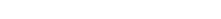, as x varies over real numbers, range over all real numbers.

## Solution

y =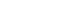or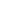+ 4yx + 3cy =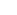+ 2x + c
or(y-1) + (4y-2)x + 3cy - c = 0
Now if we can show that the discriminant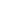for all y then for all real y there exist a real x.
Now, discriminant is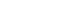- 4(y-1)(3cy-c)
We need to show- 4(y-1)(3cy-c) > 0 for any 0 < c < 1. (16-12c)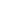- (16-16c)y + (4-4c) > 0.
This is parabola opening upword.
Now if its discriminant < 0 then this equation is always > 0.
So this is equivalent to prove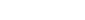- 4 (16-12c)(4-4c) < 0.
or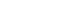- (4-3c)(1-c) < 0
or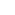-C < 0

Now given 1>c>0 so<c

or-c < 0

Conclusion: So for 1>c>0 y =Range over all real number when x varies over all real number.This is a Test of Mathematics Solution Subjective 66 (from ISI Entrance). The book, Test of Mathematics at 10+2 Level is Published by East West Press. This problem book is indispensable for the preparation of I.S.I. B.Stat and B.Math Entrance.

Also visit: I.S.I. & C.M.I. Entrance Course of Cheenta

## Problem

If c is a real number with 0 < c < 1, then show that the values taken by the function y =, as x varies over real numbers, range over all real numbers.

## Solution

y =or+ 4yx + 3cy =+ 2x + c
or(y-1) + (4y-2)x + 3cy - c = 0
Now if we can show that the discriminantfor all y then for all real y there exist a real x.
Now, discriminant is- 4(y-1)(3cy-c)
We need to show- 4(y-1)(3cy-c) > 0 for any 0 < c < 1. (16-12c)- (16-16c)y + (4-4c) > 0.
This is parabola opening upword.
Now if its discriminant < 0 then this equation is always > 0.
So this is equivalent to prove- 4 (16-12c)(4-4c) < 0.
or- (4-3c)(1-c) < 0
or-C < 0

Now given 1>c>0 so<c

or-c < 0

Conclusion: So for 1>c>0 y =Range over all real number when x varies over all real number.

### Knowledge Partner